Ex 9.4

Chapter 9 Class 12 Differential Equations
Serial order wise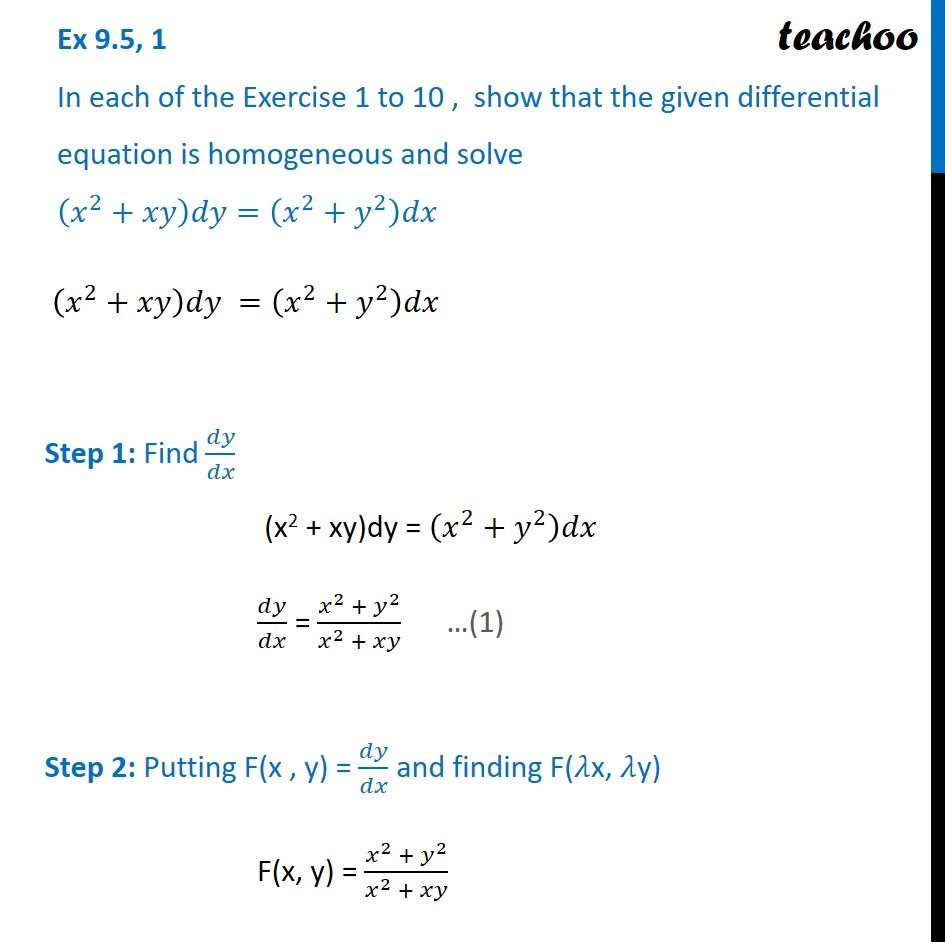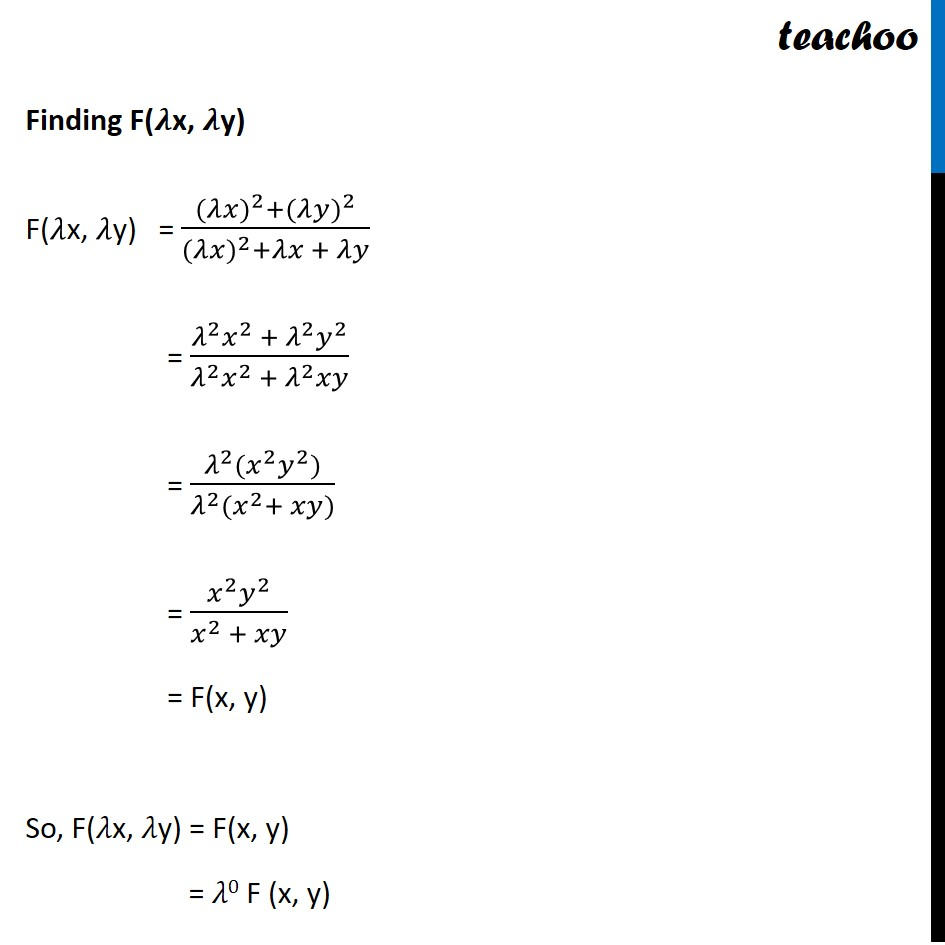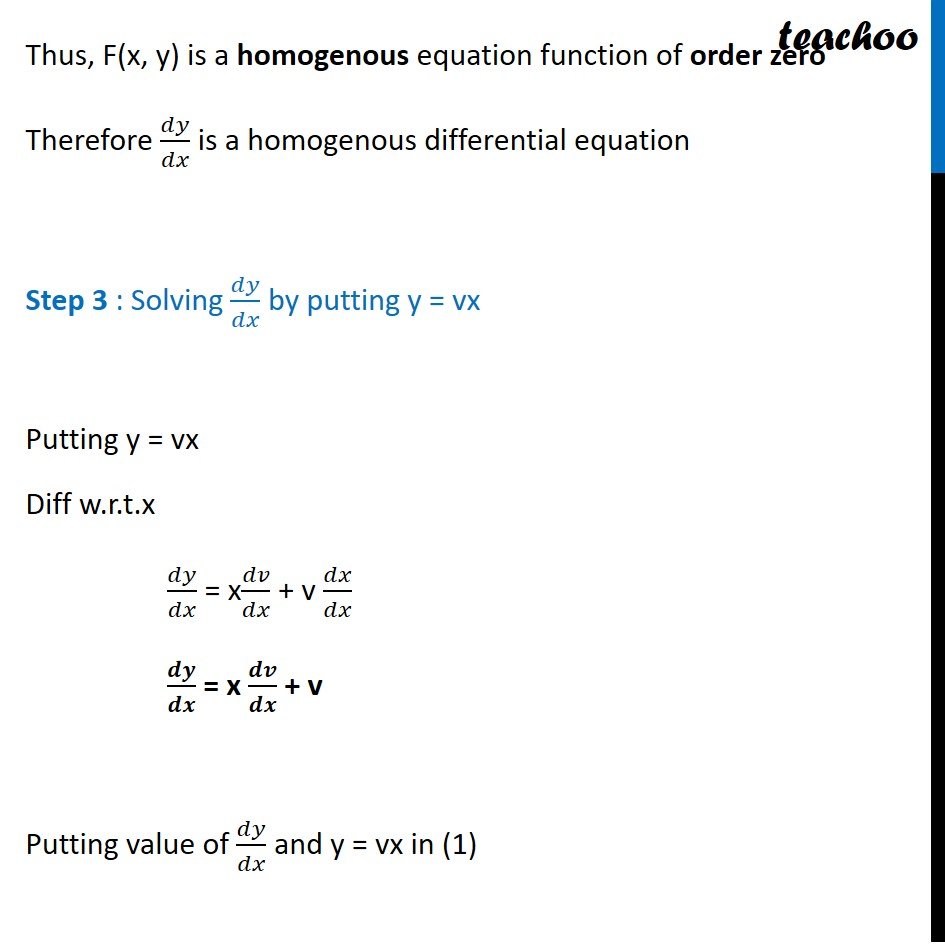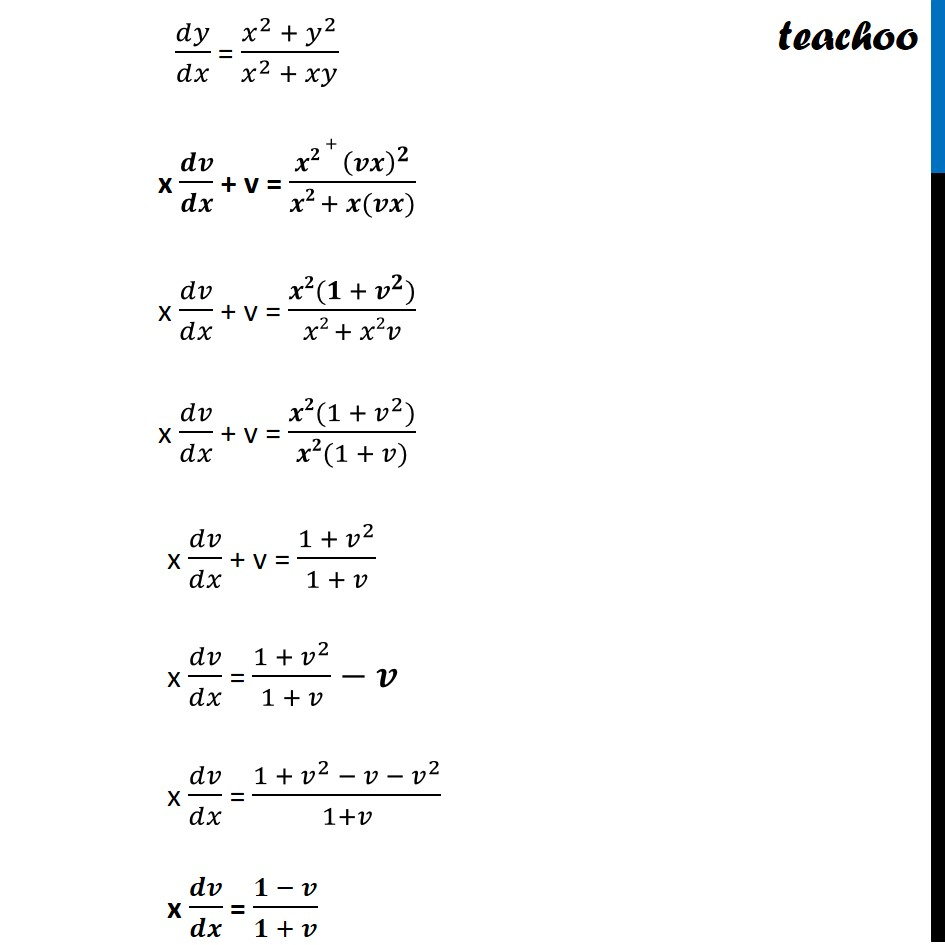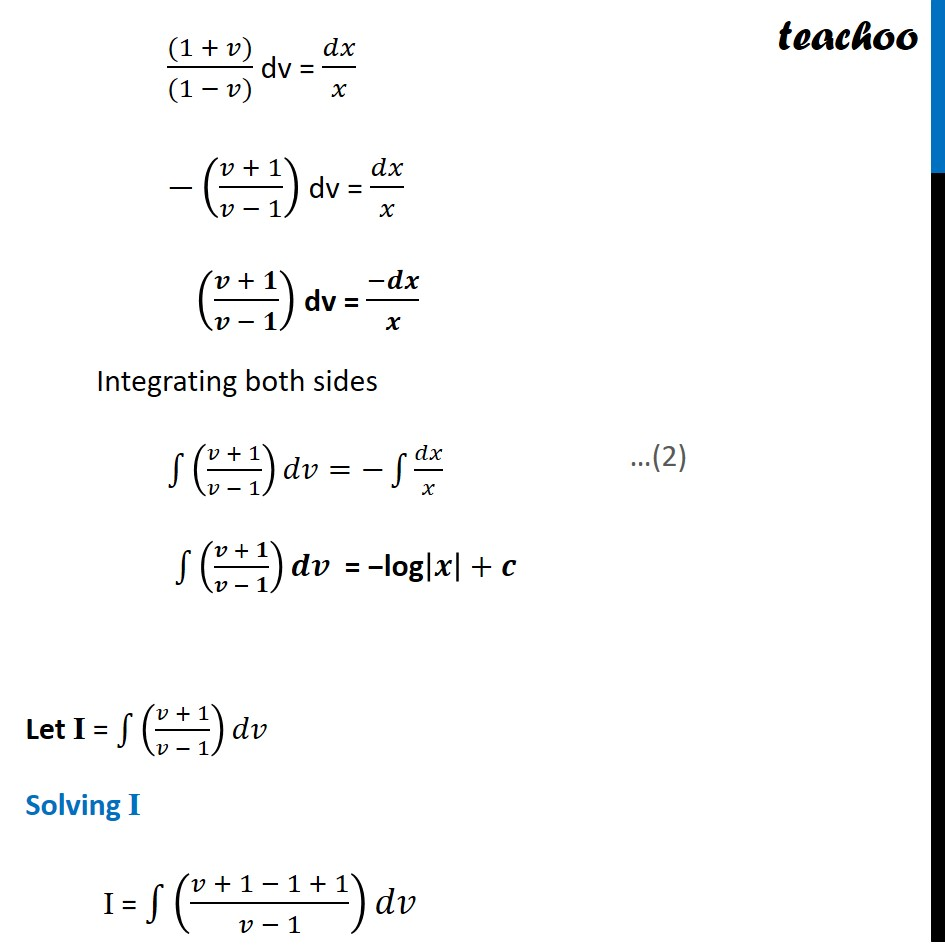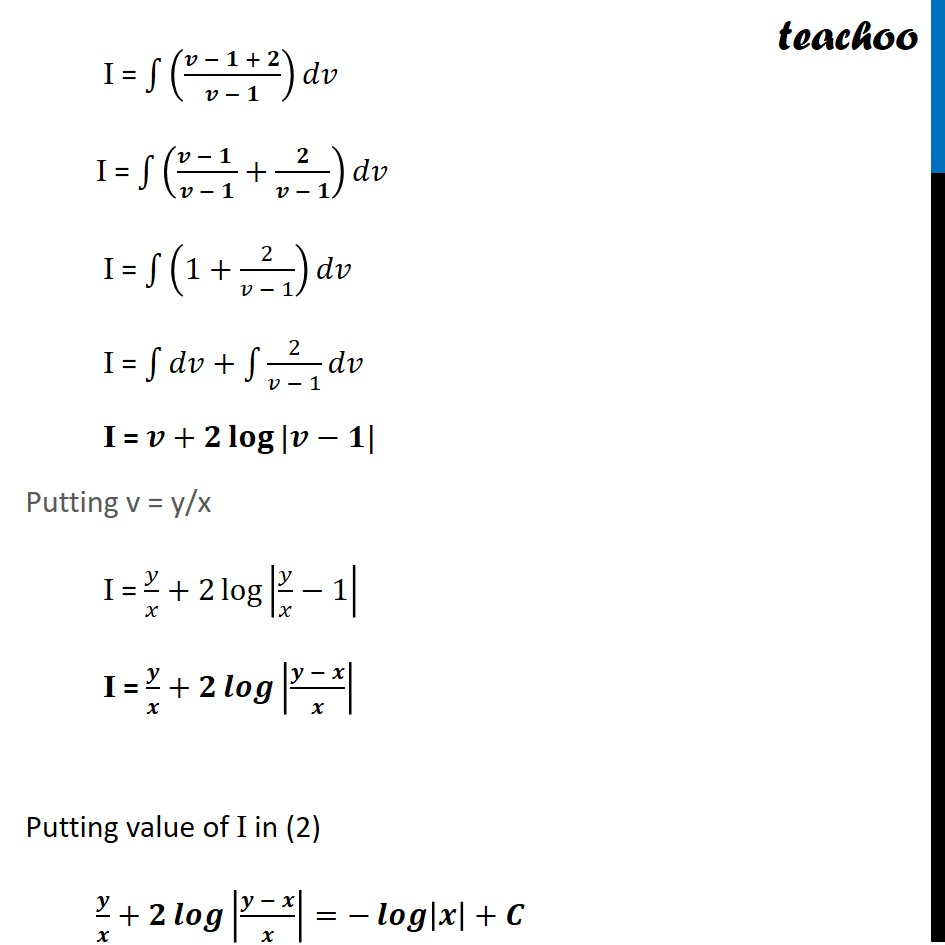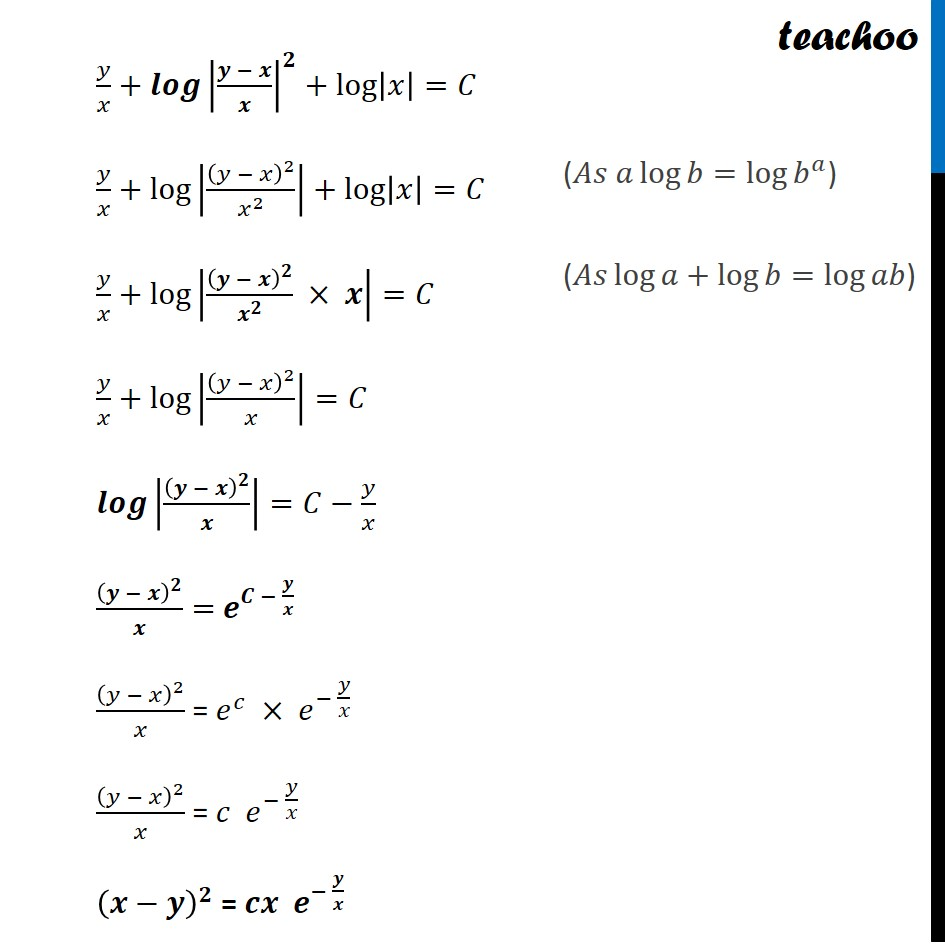Learn in your speed, with individual attention - Teachoo Maths 1-on-1 Class

### Transcript

Ex 9.4, 1 In each of the Exercise 1 to 10 , show that the given differential equation is homogeneous and solve (𝑥^2+𝑥𝑦)𝑑𝑦=(𝑥^2+𝑦^2 )𝑑𝑥 (𝑥^2+𝑥𝑦)𝑑𝑦 =(𝑥^2+𝑦^2 )𝑑𝑥 Step 1: Find 𝑑𝑦/𝑑𝑥 (x2 + xy)dy = (𝑥^2+𝑦^2 )𝑑𝑥 𝑑𝑦/𝑑𝑥 = (𝑥^2 + 𝑦^2)/(𝑥^2 + 𝑥𝑦) Step 2: Putting F(x , y) = 𝑑𝑦/𝑑𝑥 and finding F(𝜆x, 𝜆y) F(x, y) = (𝑥^2 + 𝑦^2)/(𝑥^2 + 𝑥𝑦) Finding F(𝝀x, 𝝀y) F(𝜆x, 𝜆y) = ((𝜆〖𝑥)〗^2+(𝜆〖𝑦)〗^2)/((𝜆〖𝑥)〗^2+𝜆𝑥 + 𝜆𝑦) = (𝜆^2 𝑥^2 + 𝜆^2 𝑦^2)/(𝜆^2 𝑥^2 + 𝜆^2 𝑥𝑦) = (𝜆^2 (𝑥^2 𝑦^2))/(𝜆^2 (𝑥^2+ 𝑥𝑦)) = (𝑥^2 𝑦^2)/(𝑥^2 + 𝑥𝑦) = F(x, y) So, F(𝜆x, 𝜆y) = F(x, y) = 𝜆0 F (x, y) Thus, F(x, y) is a homogenous equation function of order zero Therefore 𝑑𝑦/𝑑𝑥 is a homogenous differential equation Step 3 : Solving 𝑑𝑦/𝑑𝑥 by putting y = vx Putting y = vx Diff w.r.t.x 𝑑𝑦/𝑑𝑥 = x𝑑𝑣/𝑑𝑥 + v 𝑑𝑥/𝑑𝑥 𝒅𝒚/𝒅𝒙 = x 𝒅𝒗/𝒅𝒙 + v Putting value of 𝑑𝑦/𝑑𝑥 and y = vx in (1) 𝑑𝑦/𝑑𝑥 = (𝑥^2 + 𝑦^2)/(𝑥^2 + 𝑥𝑦) x 𝒅𝒗/𝒅𝒙 + v = (𝒙𝟐 + (𝒗𝒙)^𝟐)/(𝒙𝟐 + 𝒙(𝒗𝒙)) x 𝑑𝑣/𝑑𝑥 + v = (𝒙𝟐(𝟏 + 𝒗^𝟐))/(𝑥2 + 𝑥2𝑣) x 𝑑𝑣/𝑑𝑥 + v = (𝒙𝟐(1 + 𝑣^2))/(𝒙𝟐(1 + 𝑣)) x 𝑑𝑣/𝑑𝑥 + v = (1 + 𝑣^2)/(1 + 𝑣) x 𝑑𝑣/𝑑𝑥 = (1 + 𝑣^2)/(1 + 𝑣)−𝒗 x 𝑑𝑣/𝑑𝑥 = (1 + 𝑣^2 − 𝑣 − 𝑣^2)/(1+𝑣) x 𝒅𝒗/𝒅𝒙 = (𝟏 − 𝒗)/(𝟏 + 𝒗) ((1 + 𝑣))/((1 − 𝑣)) dv = 𝑑𝑥/𝑥 −((𝑣 + 1)/(𝑣 − 1)) dv = 𝑑𝑥/𝑥 ((𝒗 + 𝟏)/(𝒗 − 𝟏)) dv = (−𝒅𝒙)/𝒙 Integrating both sides ∫1▒((𝑣 + 1)/(𝑣 − 1)) 𝑑𝑣=−∫1▒𝑑𝑥/𝑥 ∫1▒((𝒗 + 𝟏)/(𝒗 − 𝟏)) 𝒅𝒗 = −log|𝒙|+𝒄 Let I = ∫1▒((𝑣 + 1)/(𝑣 − 1)) 𝑑𝑣 Solving I I = ∫1▒((𝑣 + 1 − 1 + 1)/(𝑣 − 1)) 𝑑𝑣 I = ∫1▒((𝒗 − 𝟏 + 𝟐)/(𝒗 − 𝟏)) 𝑑𝑣 I = ∫1▒((𝒗 − 𝟏 )/(𝒗 − 𝟏)+𝟐/(𝒗 − 𝟏)) 𝑑𝑣 I = ∫1▒(1+2/(𝑣 − 1)) 𝑑𝑣 I = ∫1▒𝑑𝑣+∫1▒2/(𝑣 − 1) 𝑑𝑣 I = 𝒗+𝟐 𝐥𝐨𝐠⁡〖|𝒗−𝟏|〗 Putting v = y/x I = 𝑦/𝑥+2 log⁡|𝑦/𝑥−1| I = 𝒚/𝒙+𝟐 𝒍𝒐𝒈⁡|(𝒚 − 𝒙)/𝒙| Putting value of I in (2) 𝒚/𝒙+𝟐 𝒍𝒐𝒈⁡|(𝒚 − 𝒙)/𝒙|=−𝒍𝒐𝒈⁡|𝒙|+𝑪 𝑦/𝑥+𝒍𝒐𝒈⁡〖|(𝒚 − 𝒙)/𝒙|^𝟐 〗+log⁡|𝑥|=𝐶 𝑦/𝑥+log⁡|(𝑦 − 𝑥)^2/𝑥^2 |+log⁡|𝑥|=𝐶 𝑦/𝑥+log⁡|(𝒚 − 𝒙)^𝟐/𝒙^𝟐 × 𝒙|=𝐶 𝑦/𝑥+log⁡|(𝑦 − 𝑥)^2/𝑥|=𝐶 𝒍𝒐𝒈⁡|(𝒚 − 𝒙)^𝟐/𝒙|=𝐶−𝑦/𝑥 (𝒚 − 𝒙)^𝟐/𝒙= 𝒆^(𝑪 − 𝒚/𝒙) (𝑦 − 𝑥)^2/𝑥 = 𝑒^𝑐 × 𝑒^(− 𝑦/𝑥) (𝑦 − 𝑥)^2/𝑥 = 𝑐 𝑒^(− 𝑦/𝑥) (𝒙−𝒚)^𝟐 = 𝒄𝒙 𝒆^(− 𝒚/𝒙) (𝐴𝑠 𝑎 log⁡𝑏=log⁡〖𝑏^𝑎 〗) (𝐴𝑠 log⁡𝑎+log⁡𝑏=log⁡𝑎𝑏)